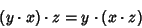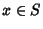## Associative Algebra

In simple terms, let,, andbe members of an Algebra. Then the Algebra is said to be associative if(1)

wheredenotes Multiplication. More formally, letdenote an-algebra, so thatis a Vector Space overand(2)(3)

Thenis said to be-associative if there exists an-D Subspaceofsuch that(4)

for alland. Here, Vector Multiplicationis assumed to be Bilinear. An-D-associative Algebra is simply said to be associative.''

Finch, S. Zero Structures in Real Algebras.'' http://www.mathsoft.com/asolve/zerodiv/zerodiv.html.